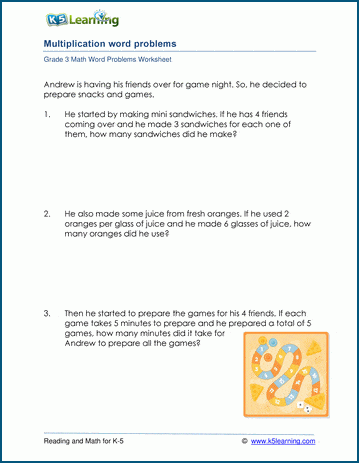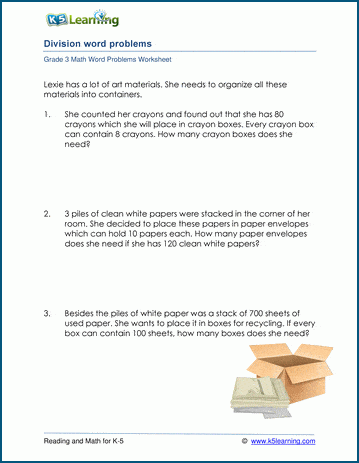# How to Know which Operations to Use in Word Problems

When working out what operation to use in a word problem, there are some key phrases students should look out for. Let’s take you through the key phrases and use them in examples.

## Key phrases for addition word problems

Find the total. How many altogether? How many are there?

For example:

There are 14 goldfish in a water tank. Kerri put in 23 more goldfish in the tank. How many goldfish are there?

Answer: 14+ 23= 37. There are 37 goldfish in the tank.

Starting in kindergarten, we’ve created addition word problems for students to practice. By grade 3, we’ve compiled mixed number word problems with addition as well.

Here’s an example of word problems of addition with sums of 50 or less from our grade 1 word problem section:## Key phrases for subtraction word problems

What is the difference? How many more? How many less?

If asked the above, the word problem involves subtraction.

For example: Mrs. Sheridan has 11cats. Mrs. Garrett has 24 cats. How many more cats does Mrs. Garrett have than Mrs. Sheridan?

Answer: 24 –11 = 13. She has 13 more cats.

## Subtraction word problem worksheets

Starting in kindergarten, we’ve created subtraction word problem for every grade. By grade 3, you’ll find subtraction included in the mixed word problems as well.

In grade 2, students work on 1-3 digit subtraction word problems.## Key phrases for multiplication word problems

How many of the same thing repeated?

If asked the above, the word problem involves multiplication.

For example: Tyler, an animal rights advocate, decided to build his own animal sanctuary to protect different animals.

500 yards away there was the aquatic reserve for freshwater organisms. If there are 6 lakes in that region, each having 175 different fish, how many fish does he have in total?

(How many groups of 175 fish are repeated across 6 lakes.)

Answer: 6 x 175 = 1,050. He has a total of 1,050 fish.

## Multiplication word problem worksheets

Starting in grade 3, students work on multiplication word problems.## Key phrases for division word problems

How many equal groups? If share evenly, how many…? The same number.

If asked the above, the word problem involves division.

For example: Sheila has started writing a list of the gifts that she plans to give to her family and friends this Christmas.

For her classmates, she made colorful paper stars which will be placed in small clear bottles. She was able to prepare 45 paper stars. How many stars will be placed in each bottle if Sheila has 9classmates?

Answer: 45 ÷ 9 = 5. There will be 5 stars placed in each bottle.

## Division word problem worksheets

Starting in grade 3, we have division word problems for students to practice.